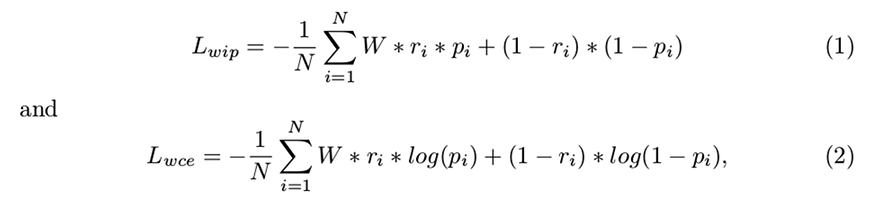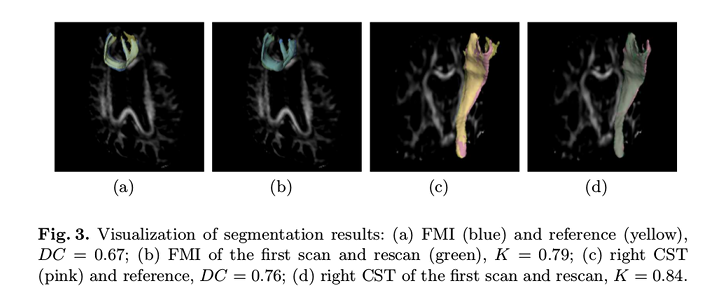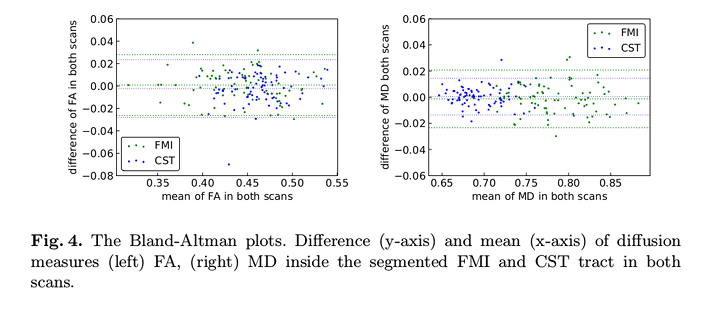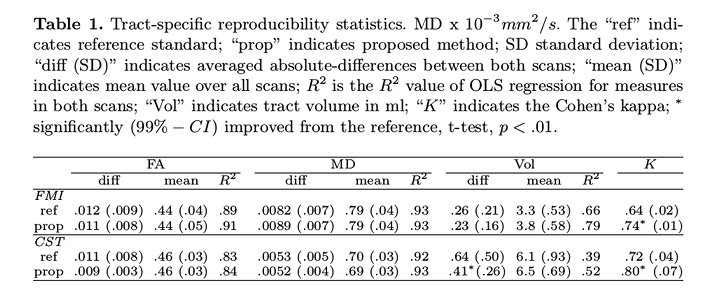# Highlights

Authors present a convolutional network to directly segment white matter tracts based on low-resolution diffusion Magnetic Resonance Imaging datasets.

# Introduction

White matter (WM) tract segmentation typically consists of a filtering process following tractography. However, authors argue that the clinical applicability of the process is limited by the fact that errors accumulate in each of the performed steps.

Authors propose to use a U-Net for WM tract segmentation based on 1 (the precursor of TractSeg) using a lower resolution dataset.

# Methods

The only modifications to the original method in 1 were adding batch normalization after each convolution layer and using PRelu activation functions instead of Relu functions.

The model outputs a binary segmentation for a specific tract.

They used a probabilistic tractography followed by tract-specific thresholding as their reference.

Segmentation accuracy was assessed by the Dice coefficient (DC).

• Input data: whether using a combination of diffusion tensor model elements, FA and MD computed from the diffusion data, voxel-wise coordinates on the MNI 152 template and T1 data provided better results.
• Loss function: whether using a weighed inner product loss function or a weighed cross-entropy function provided better results:• Class imbalance compensation: which was the tract weight that provided the best results.
• Network architecture: using two different optimizers (Adam and Nadam), whether a V-Net architecture performed better than a 3D U-Net.

The reproducibility study was based on the tract-specific median FA and MD measures.

## Data

9149 1.5 T, b = 1000 $$s/mm^2$$, 25 diffusion encoding direction diffusion Magnetic Resonance Imaging (dMRI) scans from the Rotterdam Study were used.

The reproducibility set consisted of a second round of scans of (some of) the subjects.

# Results

Authors found that:

• The diffusion tensor model elements gave the best DC results in terms of the model input data.
• The weighed inner product loss function with a fixed weight of $$W = 3$$ provided the most consistent/stable results.
• The 3D U-Net architecture using the Adam optimizer provided a better performance.

The visual results (Figure 3) show a good agreement between the proposed method and the reference, as well as between the scan and rescan data.No significant differences in the longitudinal-measures (FA, MD, volume) were found in their paired t-test, $$p > .1$$).

No consistent bias in longitudinal-measures: differences are within the $$95\%$$ limits of agreement and the mean is close to zero.The $$R^2$$ (value of ordinary least squares regression) and Cohen’s Kappa (K) (inter-rater agreement) values show that their method has better reproducibility than reference.# Conclusions

Their method compares favorably against the reference.

Their method is fast since it does not require a tractogram, and the use of low-resolution dMRI data increase its applicability to clinical practice.